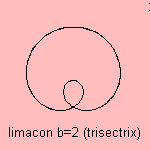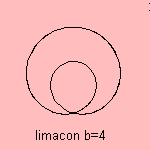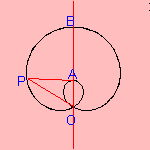# limaþon

## rouletteThe curve has the form of a snail1), so that it is also called the snail curve. It's the epitrochoid for which the rolling circle and the rolled circle have the same radius. The curve can also be defined as a conchoid. The curve is called the limaþon of Pascal (or snail of Pascal). It is not named to the famous mathematician Blaise Pascal, but to his father, Etienne Pascal. He was a correspondent for Mersenne, a mathematician who made a large effort to mediate new knowledge (in writing) between the great mathematicians of that era. The name of the curve was given by Roberval when he used the curve drawing tangents to for differentiation. But before Pascal, D³rer had already discovered the curve, since he gave a method for drawing the limaþon, in 'Underweysung der Messung' (1525). Sometimes the limaþon is confined to values b < 1. We might call this curve an ordinary limaþon. It is a transitional form between the circle (b=0) and the cardioid (b=1). When we extend the curve to values for b > 1, a noose appears.For b = 2, the curve is called the trisectrix or the limaþon trisectrix. This curve has as alternative equation: r = sin φ/3.The name of the trisectrix2) is because angle BAP is 3 times angle APO. An alternative name for the limaþon is arachnid3) or spider curve. These names follow from D³rer. Some fine properties of the curve are: the limaþon is the catacaustic and also the pedal of the circle The catacaustic quality was shown by Thomas de St Laurent in 1826 the ordinary limaþon is the inverse of the ellipse the limaþon with a noose is the inverse of the hyperbola In fact, the constant b is the same as the eccentricity for a conic section the limaþon is the orthoptic of the cardioid the curve is a special kind of botanic curve both cardioid and trisectrix are a conchoid of the circle The limaþon is an anallagmatic curve. For b unequal zero, the curve is a quartic, in Cartesian coordinates it can be written as a fourth degree equation4). notes 1) limax (Lat.) = snail 2) Tri = three, sectrix = angle. 3) from arakne (Gr.) = spider. In French: arachnÚe 4) equation: (x2 + y2 - by)2 = x2 + y2